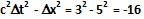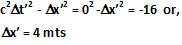## Saturday, November 21, 2009

### Irodov Problem 1.357

In Newton's view of space no matter which inertial frame we look from distance between two points in space remains the same. In other words if we have two frames K and K' with constant relative velocity w.r.t each other then.

In Einstein's world however, space shrinks as seen from the eyes of a moving observer, thus, clearly the distance between two points is not an invariant between two inertial frames. Further, time also dilates or expands for the moving observer.

It turns out that the corresponding invariant in Einstein's space-time is given byThis invariant property of Einstein's space-time can be applied to solved the problem. Let us call this invariant as the space-time distance between two events.

a) The space-time distance between events A and B as seen from the figure is given by. The space-time distance between events A and B as seen from any inertial will be the same. Thus, in a frame where the two events occur at the same point in space, we have,b) Same as above the space-time distance between events A and C is given by.

In a frame where the events occur at the same time, the distance between them will be given by